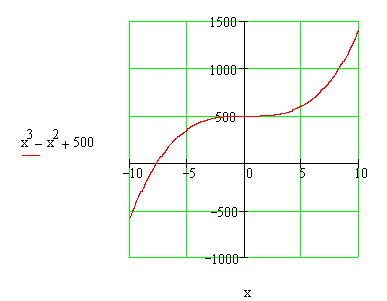Calculus on Demand at Dartmouth College Index | Lecture 2 Lecture 1Resources

Math 3 Course Syllabus
Practice Exams

Contents

In this lecture we introduce the unifying topic of the first five lectures, namely, Modeling Discrete Data with an elementary function. In particular, we discuss curve fitting by the Method of Least Squares. The material of lectures 1-5 serves as the major underpinning for our study of calculus.

Quick Question

Here is the graph of a polynomial of degree 3. Shouldn't it cross the x-axis 3 times?Textbook

Modeling Discrete Data